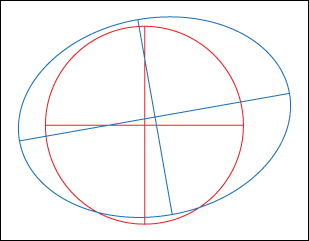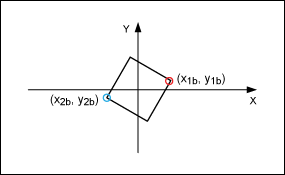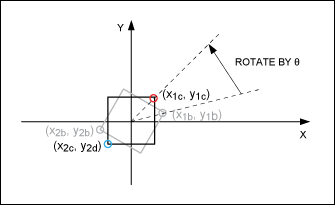# An Easy-to-Understand Explanation of Calibration in Touch-Screen Systems

### 要約

Mechanical misalignment and scaling factors lead to a mismatch between the values coming from a touch screen panel (as translated by a touch screen controller) and the display (typically an LCD) on which the touch screen panel is mounted. This tutorial discusses how to calibrate the touch screen panel to match the display.

A similar version of this article appeared in the April 20, 2012 issue of EE Times magazine.

### Introduction

Mechanical misalignment and scaling factors can lead to a mismatch between the values from a touch-panel system (in this tutorial, the touch-panel system implies a setup comprised of a touch screen and a touch-screen controller) and the display (typically an LCD) on which the touch screen is mounted. This article discusses the mathematical techniques to calibrate the touch-panel system so you can match the graphics on the display to the output from the touch-screen controller.

### Understanding Image Transformation: Translation, Rotation, and Scaling

Figure 1 is an exaggerated view of the distortion that might happen to a circle being displayed on an LCD touch-screen display. When a finger is traced around the circle (red line), the touch-panel system may give out the coordinates of an ellipse (blue line) instead of the circle, shown below in Figure 1. This change of the shape from a circle to an ellipse can be explained by the following graphic transformations: translation, rotation, and scaling.Figure 1. A circle changes shape on a touch screen because of a mismatch between the display and the touch-panel system.
Intuition suggests that any coordinate point x, y in an x-y plane that has undergone a transformation should look like:
 xNEW = f1(xOLD, yOLD) + constant1 (Eq. 1a) yNEW = f2(xOLD, yOLD) + constant2 (Eq. 1b)
Where xNEW and yNEW are the transformed coordinates; xOLD and yOLD are the old coordinates; f1() and f2() are functions that transform the old coordinates; constants1 and 2 are just that, constants.
If the transformation is linear, then functions f1() and f2() can be replaced by the following equations:
 xNEW = A xOLD + B yOLD + C (Eq. 2a) yNEW = D xOLD + E yOLD + F (Eq. 2b)
Where A, B, C, D, E, and F are constant coefficients.
Note that f1() = A xOLD +B yOLD and f2() = D xOLD + E yOLD, where constant1 and constant2 are C and F, respectively.

### The Mathematics Behind Translation, Rotation, and Scaling

An example, showing a graphic transformation, will demonstrate that the final transformation equations from this exercise will lead to the above equations 2a and 2b and, therefore, our intuition holds true. (The user can bypass this example and jump directly to the Three-Point Calibration and N-Point Calibration discussions below.)
The illustration below (Figure 2) shows a square (Shape A) that has undergone translation, rotation, and scaling (Shape B). In the process it is transformed into a rectangle.Figure 2. Translation, rotation, and scaling transform a square into a rectangle.
To rotate and scale the square (Shape A), it is important to move its center to the origin first (Figure 3) to ensure that the rotation and scaling happen uniformly.Figure 3. The square of Figure 2 (Shape A) is moved so that its center lies on the origin of the x-y axis.
This move to the center, or translation to the center, changes the value of all the coordinates of the square. For simplicity here, only the transformation of x1, y1 in Figure 2 will be explored. Consequently, x1a, y1a translates to x1b, y1b and the new equation representing this new figure is:
 x1b = x1a + Tx0 (Eq. 3a) y1b = y1a + Ty0 (Eq. 3b)
Rotate the above figure so that proper scaling can be done in the x and y directions. Figure 4 shows that the figure has been rotated counterclockwise by angle θ.Figure 4. Following Equation 3, the square is rotated counterclockwise.
The new coordinates (x1c, y1c) after the rotation are shown below. (Please see the Appendix for the derivation of the equation for this rotation.)
 x1c = x1b Cosθ - y1b Sinθ (Eq. 4a) y1c = x1b Sinθ + y1b Cosθ (Eq. 4b)
Substitute Equations 3a and 3b into Equations 4a and 4b, and simplify:
 x1c = x1a Cosθ - y1a Sinθ + Kx (Eq. 5a) y1c = x1a Sinθ + y1a Cosθ + Ky (Eq. 5b)
Where Kx = Tx0 Cosθ - Ty0 Sinθ and Ky = Tx0 Sinθ + Ty0 Cosθ.
Now scale the square in both the x and y directions to make it the same size as the rectangle in Figure 2 (Shape B). If we say that Gx is the scaling factor in the x direction and Gy is the scaling factor in the y direction, then the square will transform into a rectangle (Figure 5).Figure 5. Transform the square into a rectangle using scaling factors Gx for the x direction, and Gy for the y direction.
Multiplying Equations 5a and 5b with the scaling factors Gx and Gy yields Equations 6a and 6b, which are the coordinates for the rectangle shown in Figure 5.
 x1d = x1a Gx Cosθ - y1a Gx Sinθ + Kx Gx (Eq. 6a) y1d = x1a Gy Sinθ + y1a Gy Cosθ + Ky Gy (Eq. 6b)
Rotate the rectangle by a so that it matches the rotational orientation of Shape B in Figure 2. This leads to the transformation of (x1d, y1d) into (x1e, y1e), as shown in the Figure 6.Figure 6. The rectangle of Figure 5 is rotated so that it matches the orientation of Shape B in Figure 2.
Once again the rotation is counterclockwise. The equations for (x1e, y1e) in terms of (x1d, y1d) are:
 x1e = x1d Cosα - y1d Sinα + Kx (Eq. 7a) y1e = x1d Sinα + y1d Cosα + Ky (Eq. 7b)
Substitute Equations 6a and 6b into Equations 7a and 7b, and simplify:
 x1e = x1a A + y1a B + P (Eq. 8a) y1e = x1a D + y1a E + Q (Eq. 8b)
Where:
A = Gx Cosθ Cosα - Gy Sinθ Cosα
B = - Gx Sinθ Cosα - Gy Cosθ Cosα
P = Kx Gx Cosα - Ky Gy Sinα
D = Gx Cosθ Sinα + Gy Sinθ Cosα
E = -Gx Sinθ Sinα + Gy Cosθ Cosα
Q = Kx Gx Sinα + Ky Gy Cosα
Now a translation (Figure 7) needs to be done to move the rotated rectangle to where Shape B appears in Figure 2.Figure 7. Translation of the rectangle from the origin to the location where Shape B appears in Figure 2.
Assume that the translation in the x and y direction is Tx and Ty, respectively. Therefore, Equations 8a and 8b will change to:
 x1f = x1a A + y1a B + C (Eq. 9a) y1f = x1a D + y1a E + F (Eq. 9b)
Where C = P + Tx and F = Q + Ty.
Equations 9a and 9b are what we want—they match Equations 1a and 1b. Note that the coordinate (x1f, y1f) is in terms of (x1a, y1a). In the touch panel mounted on a display the user will have to determine the A, B, C, D, E, and F during calibration.
Please note that the purpose of this exercise is to give the user an appreciation of the general form of the equation for the transformation, shown by Equations 9a and 9b. This article shows that we will arrive at this general form irrespective of the direction and magnitude of rotation, translation, and scaling.

### Three-Point Calibration

We start with a pair of equations, the final pair in our mathematical exercise above.
 x1f = x1a A + y1a B + C (Eq. 9a) y1f = x1a D + y1a E + F (Eq. 9b)
The goal of the touch-panel system calibration is to solve the Equations 2a and 2b (or similarly Equations 9a and 9b) to derive values for A, B, C, D, E, and F.
Looking at these equations we know that there are six unknowns. Therefore, we will need six equations to solve for these unknowns and this can be achieved by doing a three-point calibration for a touch-panel system. The user will generate three pairs of (x, y) coordinates by touching the panel at the three pairs of display coordinates: (x1d, y1d), (x2d, y2d) and (x3d, y3d). If their corresponding touch-screen values (as presented by the touch-screen controller) are (x1, y1), (x2, y2), and (x3, y3), then the six unknowns can be solved by the equations shown below. These points must be independent of each other, as shown in Figure 8.Figure 8. LCD panel showing three display coordinates for the user to touch.
x1d = x1 A + y1 B + C
x2d = x2 A + y2 B + C
x3d = x3 A + y3 B + C
y1d = x1 D + y1 E + F
y2d = x2 D + y2 E + F
y3d = x3 D + y3 E + F
Now with six equations for the six unknowns, the above can be written in matrix form as:Therefore, a little matrix manipulation will yield A, B, C, D, E, and F, as shown below:Where, Z-1 is the inverse matrix of Z.

### Three-Point Calibration Example

We will use the MAX11800 touch-screen controller in this example. Assume that an LCD panel display has a resolution of 256 x 768 and that the three calibration points chosen are (65, 350), (200, 195), and (195, 550). These are the exact points that we want the touch panel to show when it is touched. However, since the resolution for the MAX11800 is 4096 x 4096 (12 bits) and because of mechanical misalignment, the coordinate will come out differently. For this exercise, we assume that these values are: (650, 2000), (2800, 1350), and (2640, 3500), respectively. Please note: these values are made up and serve as an example only.
Solving for A, B, C, D, E, and F using Equations 10a and 10b, we get the following:
A = 0.0635
B = 0.0024
C = 18.9116
D = -0.0227
E = 0.1634
F = 37.8887
Therefore, the equations that will generate the x and y coordinates for this particular example are:
xd = 0.0635 x + 0.0024 y + 18.9116
yd = -0.0227 x + 0.1634 y + 37.8887
Where (x, y) are the coordinates coming from the touch-panel system and (xd, yd) is the adjusted value.

### N-Point Calibration

The user may choose to calibrate using more points. A generalized form of the sets of equations will look like the following:
 x1d = x1 A + y1 B + C x2d = x2 A + y2 B + C x3d = x3 A + y3 B + C ... xnd = xn A + yn B + C (Eq. set 11a)
 y1d = x1 D + y1 E + F y2d = x2 D + y2 E + F y3d = x3 D + y3 E + F ... ynd = yn D + y3 E + F (Eq. set 11b)
Where, (x1d, y1d)...(xnd, ynd) are coordinates generated by the display; (x1, y1)...(xn, yn) are the corresponding values (for n points) determined by the MAX11800 from the touch-screen panel. The goal is to determine the coefficients using these values.
In the Equation set 11a there are three unknowns, A, B, and C, but the set of coordinates is more than three. This implies that there are more equations than unknowns. Therefore, in this instance it makes sense to use the least square fit to utilize all the points and derive an average value of the coefficients. This also means that more calibration points would help lower errors. The least square fit is represented in Figure 9. The same technique will be applied for determining the unknowns D, E, and F for the y values.Figure 9. An average value for the coefficients A, B, and C is found by applying the least square fit to the points.
Equation sets 11a and 11b can be written in matrix form, as shown:By using the least square fit in this matrix form, the coefficients are given by the equations below. (Please refer to a book on regression analysis for the derivation of the matrix form of the least square fit form.)### N-Point Calibration Example

Once again, assume that an LCD panel display has a resolution of 256 x 768 and the five calibration points are (100, 350), (50, 200), (200, 200), (210, 600), and (65, 600). These are the exact points that we want the touch panel to show when it is touched. However, since the resolution for the MAX11800 is 4096 x 4096 (12 bits) and because of mechanical misalignment, the points may come out differently. Again assume that they are (1700, 2250), (750, 1200), (3000, 1500), (2500, 3400), and (600, 3000), respectively. Please note: these values are made up and meant to serve as an example only.
Solving for A, B, C, D, E, and F using Equations 12a and 12b, we get:
A = 0.0677
B = 0.0190
C = -33.7973
D = -0.0347
E = 0.2100
F = -27.4030
Therefore, the equations to generate the x and y coordinates for this particular example are:
xd = 0.0677 x + 0.0190 y - 33.7973
yd = -0.0347 x + 0.2100 y - 27.4030
Where (x, y) are the coordinates coming from the touch-panel controller, and (xd, yd) is the adjusted coordinate value to match what is shown on the display.

### Example Summary

If the least squares technique is used on three points, it will yield the same coefficient as the three-point calibration. It is mathematically easier, therefore, to handle the three-point calibration as three simultaneous linear equations with three unknowns instead of the lengthier calculation with the least squares technique.

### Appendix: Derivation of the Equation for a Counterclockwise RotationThe original point (x, y) can be written in polar form as:
 x = r Cosθ and y = r Sinθ, (Eq. A)
Where r is the radial distance from the origin to the (x, y) coordinate.
After the rotation the (x, y) coordinates become (x', y'):
 x' = r Cos(θ + Φ) and y' = r Sin(θ + Φ) (Eq. B)
Using the trig identities below:
 Cos(θ + Φ) = CosΦ Cosθ - SinΦ Sinθ Sin(θ + Φ) = SinΦ Cosθ - CosΦ Sinθ (Eq. C)
And substituting in Equation B, we get:
 x' = r CosΦ Cosθ - r SinΦ Sinθ y' = r SinΦ Cosθ - r CosΦ Sinθ (Eq. D)
Substituting Equation A into Equation D:
 x' = x CosΦ - y SinΦ y' = x SinΦ - y CosΦ (Eq. E)
The derivation for the clockwise rotation is left as an exercise for the user.